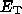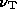# Introduction

Radio galaxies and radio quasars emit radio waves as synchrotron radiation from relativistic electrons spiralling in a magnetic field. At least in the radio lobes, where the source is optically thin at most observing frequencies, the radio spectrum can often be described by a power law over a reasonable range of frequencies, and it is often assumed that both the original radio spectrum and the distribution of electron energies was a power law. Energy losses, most pronounced at high electron energies, cause the spectrum to deviate from its initial shape, causing a steepening of the high-frequency spectrum (eg. Myers & Spangler 1985; Tribble 1993, hereafter Paper I). The two standard models assume either that electron pitch angles remain constant (the KP model - Kardashev 1962; Pacholczyk 1970), or that the pitch angles are rapidly isotropized by scattering (the JP model - Jaffe & Perola 1973).

The difference in pitch angle behaviour between the simple KP and JP models leads to substantial differences in the resulting synchrotron spectra. In the JP model, each electron has sampled all possible pitch anglesbecause its pitch angle has been continually randomized by the scattering. The total losses are then the instantaneous losses B^2sin^2, averaged over all pitch angles to give B^2<sin^2>, and are independent of an electron's current pitch angle. There is a sharp cutoff in the energy spectrum at a break energythat is independent of pitch angle or viewing orientation. The resulting spectrum is sharply cut off at a frequency= c_1 B^2 sinwhere B is the field strength and c_1 is a constant (Pacholczyk 1970). There is little variation of the spectrum with viewing angle so that averaging over different orientations of the field gives a total spectrum that retains the sharp cutoff.

In the KP model there is still a sharp break in the energy spectrum at a given pitch angle, but the break energydepends on the pitch anglebecause the losses are strongly dependent on pitch angle - the sin^2factor is not averaged away. At a given field orientation, the spectrum is sharply cut off. Integrating over all field orientations eliminates the sharp cutoff in the spectrum because the total spectrum includes contributions from electrons with all pitch angles, which have a wide range of break frequencies. In particular, the electrons with very small pitch angles have very low losses and a correspondingly highand, so that while at high frequencies the radiation from the electrons with large pitch angles has faded, we still see the emission from the electrons travelling at very small angles to the field.

The JP model has a much more secure physical basis than the KP model. The electron pitch angle distribution in the KP model is anisotropic - such a pitch angle distribution is unstable as it excites Alfvén waves which scatter the electrons back to an isotropic pitch angle distribution (Wentzel 1969; Melrose 1980). Inverse Compton scattering, variations in field strength and direction, and any waves generally present in the relativistic radio plasma will also isotropize the electron pitch angle distribution. Thus the JP model should give a much better description of aged radio spectra than the KP model. Unfortunately, detailed multifrequency observations of Cygnus A (Carilli et al. 1991) supported the KP model, not finding the extremely strong break in the spectrum predicted by the JP model.

Furthermore, Katz-Stone, Rudnick & Anderson (1993) analysed the Cygnus A spectra and questioned the assumption that the spectral shape was due to ageing of an underlying power law spectrum. They introduced the concept of the radio colour-colour plot and proposed that it could be a useful diagnostic tool for studying radio spectra. They showed that the traditional radio spectral models (specifically the simple JP and KP models) do not give a good description of the spectrum of Cygnus A, and argued that the spectral shape was not solely due to ageing effects. If this is true, it would require a radical revision of the standard assumptions used in spectral ageing analyses.

However, realistic models of the ageing process give spectra with a variety of shapes (see Paper I). These models allow for the effects of a spatially varying magnetic field, inverse Compton losses, and diffusion of electrons between regions of different field strength. The general effect is to smooth out the sharp spectral break of the JP model by superposing the spectra from regions where the physical conditions cause the break frequency to differ. The variation in break frequency is caused by spatial variations in energy losses which set the energyat which the energy spectrum breaks, and by variations in magnetic field strength which convert the break energyinto the corresponding break frequency.

In this paper I present theoretical colour-colour diagrams for a variety of realistic spectra such as those presented in Paper I, and compare them to the particular case of Cygnus A.

Up to: ___________________________________
Peter Tribble, peter.tribble@gmail.com